# Some interesting rotation sequences

We mention here two simple, but intriguing, sequences of rotations that are featured in the Hamilton-Rodrigues theorem and in Codman’s paradox. The compound rotation associated with the latter can be visualized with a sequence of arm motions.

## The Hamilton-Rodrigues theorem

The Hamilton-Rodrigues theorem, which dates to Rodrigues  and Hamilton  in the mid-19th century, states that a sequence of 180rotations about three mutually perpendicular axes is equivalent to the identity transformation. To prove this theorem, we first let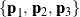be a set of right-handed orthonormal basis vectors and then use Euler’s representation of a rotation to see that, for a rotation of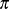rad about each axis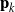,

(1)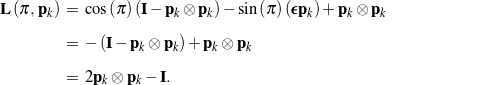The sequence of rotations of interest is illustrated in Figure 1:, then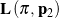, followed by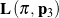. That is, the compound rotation is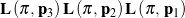.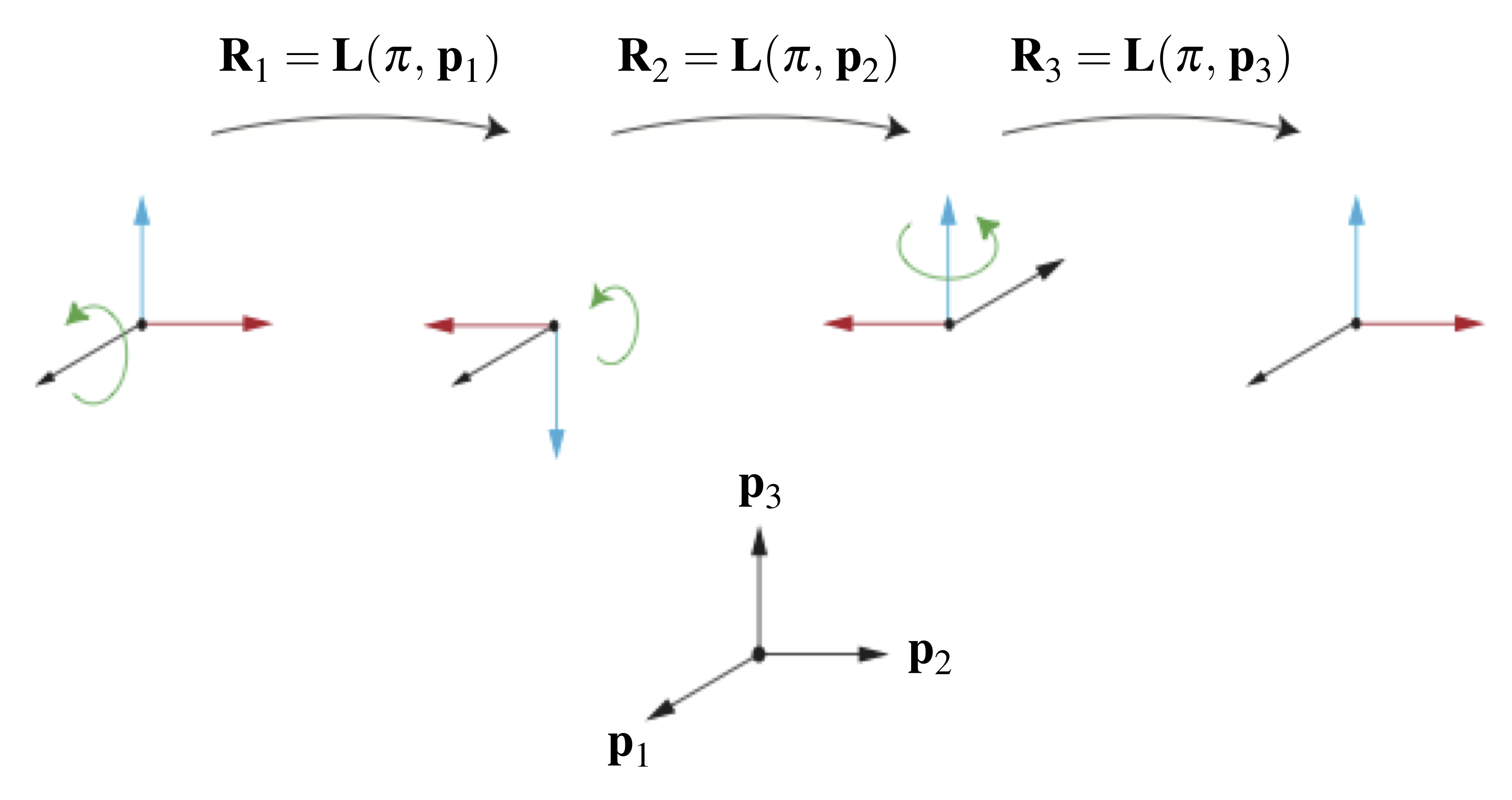Figure 1. Illustration of the Hamilton-Rodrigues theorem, which states that the composition of three rotations of rad about three mutually perpendicular axes is equivalent to the identity transformation.

With the help of (1), a direct computation shows that the compound rotation

(2)which proves the theorem. An alternative approach to proving the Hamilton-Rodrigues theorem is presented by Whittaker in Section 3 of , in which he uses a purely geometric proof that differs from our algebraic approach above. As shown by Ziegler  and further discussed in , the Hamilton-Rodrigues theorem can be used to show that a constant moment is not conservative. Additional discussions of conservative moments and moment potentials can be found in [6, 7, 8, 9]. We can also use the theorem to show that a pair of 180rotations about two perpendicular axes, say,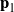and, is equivalent to a rotation of 180about an axis that is mutually perpendicular:

(3)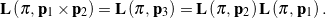Codman’s paradox features the rotation of the arm about the shoulder joint and dates to 1934 . As quoted from Politti et al. , to observe Codman’s paradox, first place your right arm hanging down along your side with your thumb pointing forward and your fingers pointing toward the ground. Next, elevate your arm horizontally so that your fingers point to the right, and then rotate your arm in the horizontal plane so that your fingers now point forward. Finally, rotate your arm downward so that your fingers eventually point toward the ground. After these three rotations, you will notice that your thumb points to the left. That is, your arm rotated by 90. The fact that you get this rotation without having performed a rotation about the longitudinal axis of your arm is known as Codman’s paradox. The sequence of rotations we just described is depicted in Figure 2.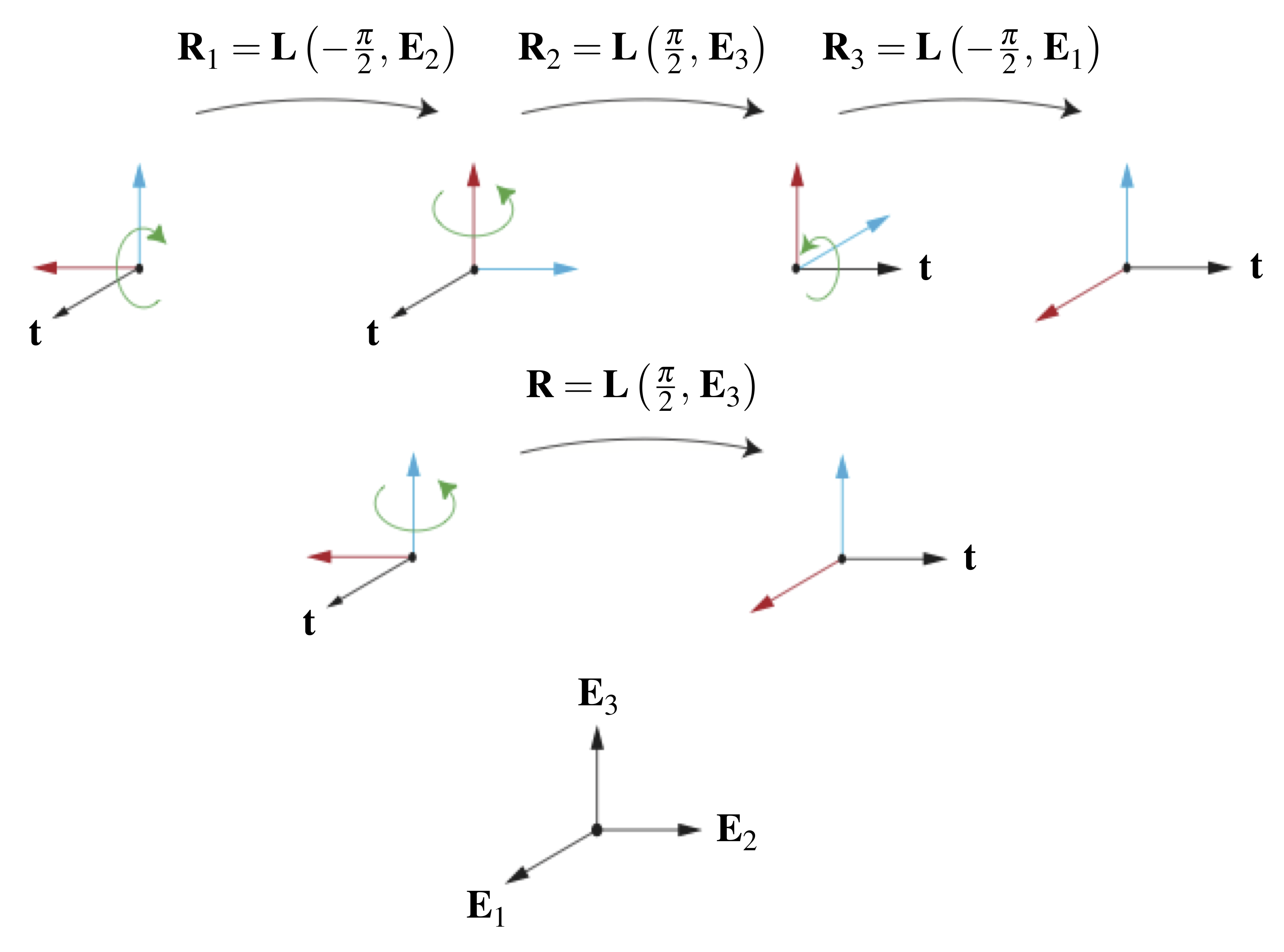Figure 2. Representation of the three rotations featuring in Codman’s paradox. The vector corresponds to the longitudinal axis of the right thumb.

To explain the 90rotation observed in Codman’s paradox, we first define a right-handed orthonormal basis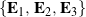for which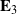points up,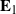points to the right, and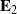points forward, as shown in Figure 2. The first rotation discussed above is a clockwise rotation of 90about:

(4)The second rotation is a 90counterclockwise rotation about:

(5)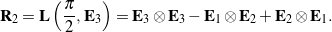Lastly, the third rotation is a clockwise rotation of 90about:

(6)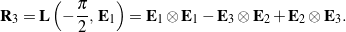Consequently, the compound rotation

(7)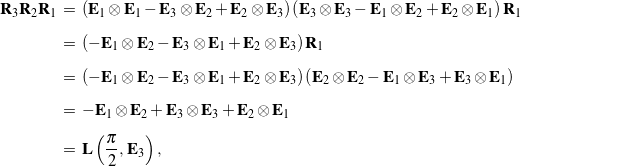or, equivalently,

(8)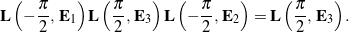Thus, the sequence of rotations featured in Codman’s paradox is equivalent to a single counterclockwise rotation about the vertical axisby 90, implying that there is no apparent contradiction in Codman’s observations on the behavior of the shoulder joint. This result has an interpretation that can be used to show that successive rotations about three perpendicular axes can be reproduced by a single rotation about one of the axes. A second explanation for the 90rotation in the motion of the arm discussed earlier is to follow  and parameterize the rotation using a set of Euler angles,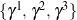. In this case, when the Euler basis vectorreturns to its initial orientation,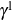and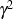also return to their initial values, and the relative rotation is the given by the change in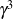. It is straightforward to show that this change is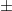90. Additional examples of using Euler angles to examine relative rotations can be found in .

An extraordinary geometric explanation of Codman’s paradox can be found in Section 123 of Kelvin and Tait’s remarkable Treatise on Natural Philosophy that was first published in 1865 (the same section can be found in the reprinted edition ). Kelvin and Tait’s developments were subsequently rediscovered nearly a century later  and feature in modern works on geometric phases (see [12, 15] and references therein). Referring to the left-hand panel in Figure 3, consider a unit vector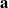that corotates with a rigid body, and suppose the motion of the body is such thateventually returns to its original orientation. The locus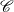ofon the unit sphere encloses a region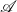on the sphere’s surface. Kelvin and Tait’s theorem states that, modulo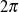, the amount the body has rotated aboutis equal to the surface area of.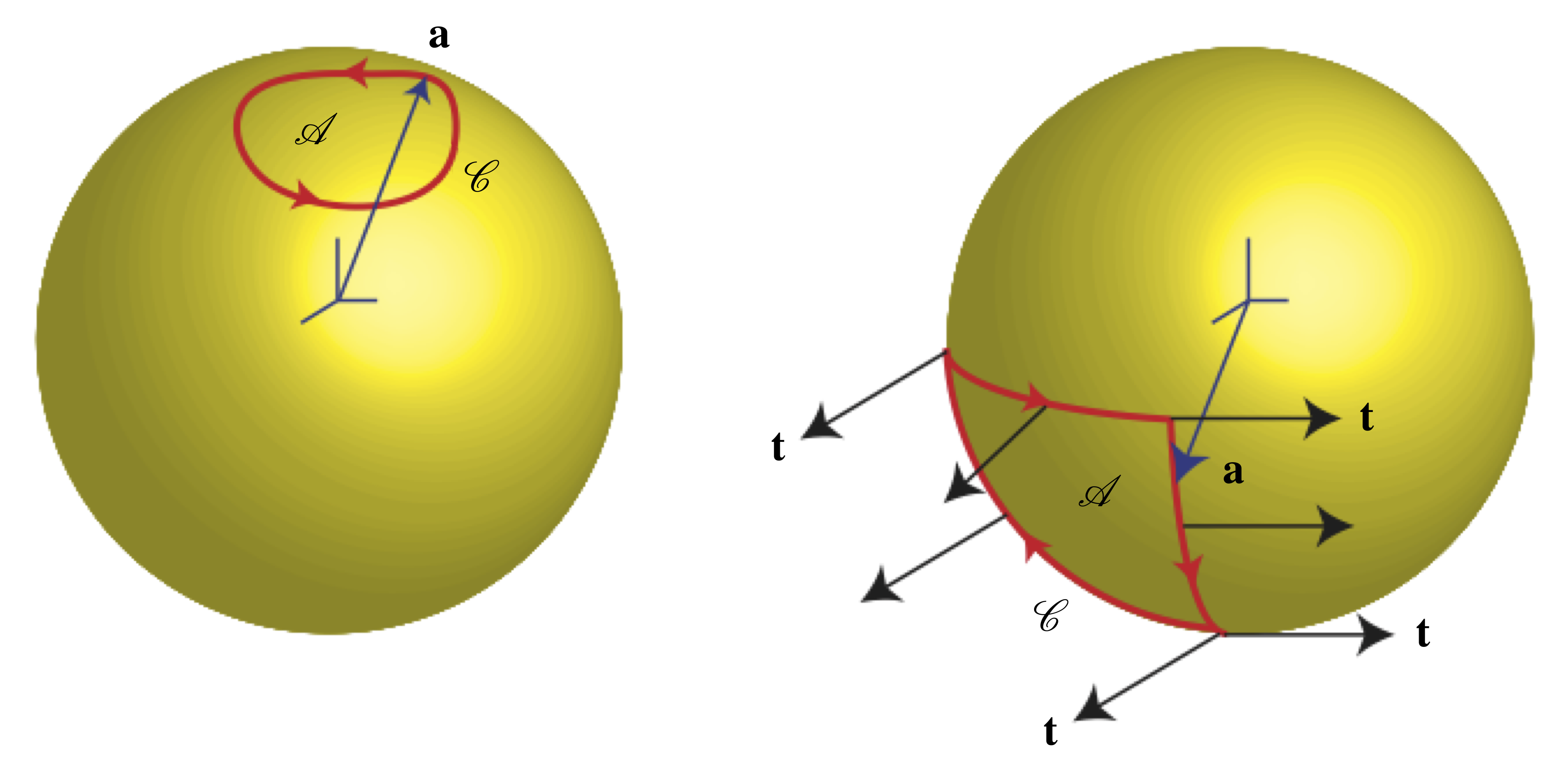Figure 3. Loci of a unit vector on the surface of the unit sphere. The panel on the left is used to elucidate Kelvin and Tait’s theorem on relative rotation. The panel on the right shows the locus of for the arm motion featured in Codman’s paradox, where points along and corotates with the longitudinal axis of the arm.

To apply Kelvin and Tait’s theorem to examine Codman’s paradox, we refer to the right-hand panel in Figure 3 and imagine the shoulder joint as fixed at the origin of the unit sphere. We trace the locus of, which points from the origin to the finger tips and corotates with the arm. During the arm motion featured in Codman’s paradox, the tip oftraces out a spherical triangle on the unit sphere’s surface. The surface area of this triangle occupiesth of the sphere’s total surface area, which is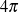. Therefore, the surface area of the triangle is, which is identical to the angle of rotation for the compound rotation given in (8).